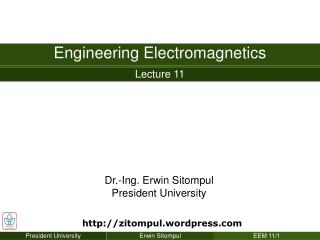DownloadDownload PresentationAmpere’s Circuital Law

# Ampere’s Circuital Law

Télécharger la présentation## Ampere’s Circuital Law

- - - - - - - - - - - - - - - - - - - - - - - - - - - E N D - - - - - - - - - - - - - - - - - - - - - - - - - - -
##### Presentation Transcript

1. Chapter 8 The Steady Magnetic Field Ampere’s Circuital Law • In solving electrostatic problems, whenever a high degree of symmetry is present, we found that they could be solved much more easily by using Gauss’s law compared to Coulomb’s law. • Again, an analogous procedure exists in magnetic field. • Here, the law that helps solving problems more easily is known as Ampere’s circuital law. • The derivation of this law will waits until several subsection ahead. For the present we accept Ampere’s circuital law as another law capable of experimental proof. • Ampere’s circuital law states that the line integral of magnetic field intensity H about any closed path is exactly equal to the direct current enclosed by that path,

2. Chapter 8 The Steady Magnetic Field Ampere’s Circuital Law • The line integral of H about the closed path a and b is equal to I • The integral around path c is less than I. • The application of Ampere’s circuital law involves finding the total current enclosed by a closed path.

3. Chapter 8 The Steady Magnetic Field Ampere’s Circuital Law • Let us again find the magnetic field intensity produced by an infinite long filament carrying a current I. The filament lies on the z axis in free space, flowing to az direction. • We choose a convenient path to any section of which H is either perpendicular or tangential and along which the magnitude H is constant. • The path must be a circle of radius ρ, and Ampere’s circuital law can be written as

4. Chapter 8 The Steady Magnetic Field Ampere’s Circuital Law • As a second example, consider an infinitely long coaxial transmission line, carrying a uniformly distributed total current I in the center conductor and –I in the outer conductor. • A circular path of radius ρ, where ρ is larger than the radius of the inner conductor a but less than the inner radius of the outer conductor b, leads immediately to • If ρ < a, the current enclosed is • Resulting

5. Chapter 8 The Steady Magnetic Field Ampere’s Circuital Law • If the radius ρ is larger than the outer radius of the outer conductor, no current is enclosed and • Finally, if the path lies within the outer conductor, we have • ρ components cancel,z component is zero. • Only φ component of H does exist.

6. Chapter 8 The Steady Magnetic Field Ampere’s Circuital Law • The magnetic-field-strength variation with radius is shown below for a coaxial cable in which b = 3a, c = 4a. • It should be noted that the magnetic field intensity H is continuous at all the conductor boundaries  The value of Hφ does not show sudden jumps. • Outside the coaxial cable, a complete cancellation of magnetic field occurs. Such coaxial cable would not produce any noticeable effect to the surroundings (“shielding”)

7. Chapter 8 The Steady Magnetic Field Ampere’s Circuital Law • As final example, consider a sheet of current flowing in the positive y direction and located in the z = 0 plane, with uniform surface current density K = Kyay. • Due to symmetry, H cannot vary with x and y. • If the sheet is subdivided into a number of filaments, it is evident that no filament can produce an Hy component. • Moreover, the Biot-Savart law shows that the contributions to Hz produced by a symmetrically located pair of filaments cancel each other.  Hz is zero also. • Thus, only Hx component is present.

8. Chapter 8 The Steady Magnetic Field Ampere’s Circuital Law • We therefore choose the path 1-1’-2’-2-1 composed of straight-line segments which are either parallel or perpendicular to Hx and enclose the current sheet. • Ampere's circuital law gives • If we choose a new path 3-3’-2’-2’3, the same current is enclosed, giving and therefore

9. Chapter 8 The Steady Magnetic Field Ampere’s Circuital Law • Because of the symmetry, then, the magnetic field intensity on one side of the current sheet is the negative of that on the other side. • Above the sheet while below it • Letting aN be a unit vector normal (outward) to the current sheet, this result may be written in a form correct for all z as

10. Chapter 8 The Steady Magnetic Field Ampere’s Circuital Law • If a second sheet of current flowing in the opposite direction, K = –Kyay, is placed at z = h, then the field in the region between the current sheets is and is zero elsewhere

11. Chapter 8 The Steady Magnetic Field Ampere’s Circuital Law • The difficult part of the application of Ampere’s circuital law is the determination of the components of the field which are present. • The surest method is the logical application of the Biot-Savart law and a knowledge of the magnetic fields of simple form (line, sheet of current, “volume of current”). • Solenoid • Toroid

12. Chapter 8 The Steady Magnetic Field Ampere’s Circuital Law • For an infinitely long solenoid of radius a and uniform current density Kaaφ, the result is • If the solenoid has a finite length d and consists of N closely wound turns of a filament that carries a current I, then

13. Chapter 8 The Steady Magnetic Field Ampere’s Circuital Law • For a toroid with ideal case • For the N-turn toroid, we have the good approximations

14. Chapter 8 The Steady Magnetic Field End of the Lecture